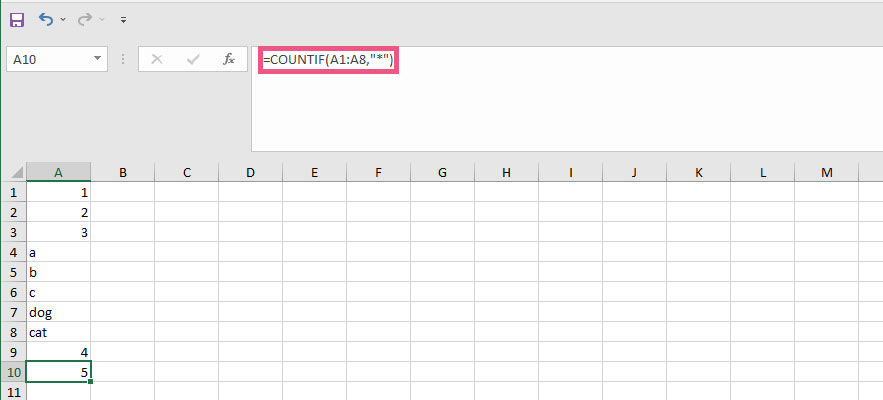# How to Count Cells with Text in Excel

Updated

Here is our step-by-step guide to teach you how to count cells that contain text instead of numbers using Microsoft Excel and Google Sheets.

Spreadsheet software like Microsoft Excel and Google Sheets is perfect for manipulating data. There are seemingly limitless possibilities for manipulating, arranging, and sifting through data using these programs. You may eventually need to do a data query to count cells containing text. If you have a mix of numbers and letters in a data set, it is possible to figure out how many of those cells contain text.

We’ll show you how to count cells with text in Excel and Google Sheets in the guide below. The same process applies to both programs, as they share many of the same functions and equations. No matter which program you prefer, you can follow the steps below to accomplish the task.

## How to Count Cells with Text in Excel

Here is how to count cells with text in Excel:

1. Select a cell where you want to display the count
2. Use the equation =COUNTIF
3. Select the range of data you want to include in your query
4. Enter “*” as the criteria to look for any text in the range
5. Press enter to count the cells with text

Open your data set in Microsoft Excel, and find a cell where you’d like to perform the count. Start by pressing the = sign, and use the following equation:

````=countif(range,criteria)`

```How to use countif to count cells containing text (Techozu/Microsoft Excel)

In the example above, our range is cells A1 through A8 (A1:A8), and the criteria are “*”, which means any cell containing text. The asterisk is a wildcard symbol that essentially means “any letters or words.”

As seen above, the equation correctly identified five cells containing text. The result can be seen in cell A10.

## How to Count Cells with Text in Google Sheets

Here is how to count cells with text in Google Sheets:

1. Select a cell where you want to display the count
2. Use the equation =COUNTIF
3. Select the range of data you want to include in your query
4. Enter “*” as the criteria to look for any text in the range
5. Press enter to count the cells with text

First, open your data in Google Sheets. Find a cell that’s empty where you would like to perform the count. Next, begin typing in the equation, which should look like this:

````=countif(range,criteria)`

```